729 What I Did for Paula Krieg’s Star Project

Let me tell you a little about Paula Krieg’s Star Project:

The 12-Fold Rosette has its origin in Islamic Geometry. Paula Krieg is asking that people from a variety of backgrounds color in a section of the rosette and send it back to her so that she can assemble the different pieces into one lovely unified rosette. We are all stars, and some of the pieces look like stars, so she calls it her star project. You are invited to become a part of the project as well. Just click on this invitation to learn more. You can get a tile by either leaving a comment or e-mailing her a request, but do it soon. You will need to return your tile to her by 10 January 2016 to be included in the project.Rosette from Paula Krieg’s blog showing some possible coloring schemes for the tiles.

The piece that Paula sent me looked like this:I thought I would color it black, gray, white, and red because I think they look good together.

Since I don’t have any crayons in my house right now, and all my coloring pencils need to be sharpened, I decided to color it on my computer using the program Paint.

I brought up the e-mail Paula, opened the attachment, hit “Print Screen”, copied and pasted it into Paint, then cropped it.

I soon discovered that I could color in my entire tile using only black and gray and stay true to a goal I had given myself. The very tiny triangles insisted on staying white, but if I had increased the view size before I hit “Print Screen”, I would have been able to color them, too.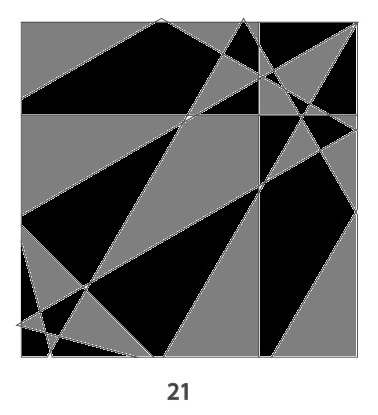I saved my coloring, but immediately felt like it really should have some red in it. I brought up my saved work, clicked on “save as”, named it “red tile”, and added some red. However, saving the file changed how it now received color. When I added some red, it looked like this, and I liked it and saved it again: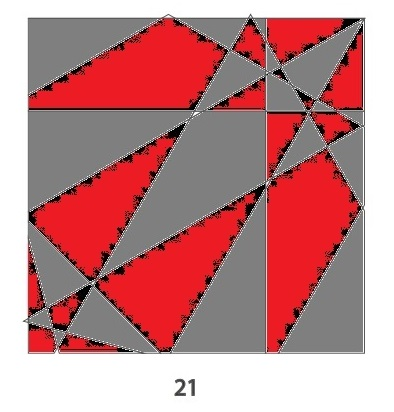Then I thought I might want a little more white, so I brought it back up and did “save as” again before adding some. This time it seem to allow even less additional color in each shape. (In other words, we see much more gray than black.)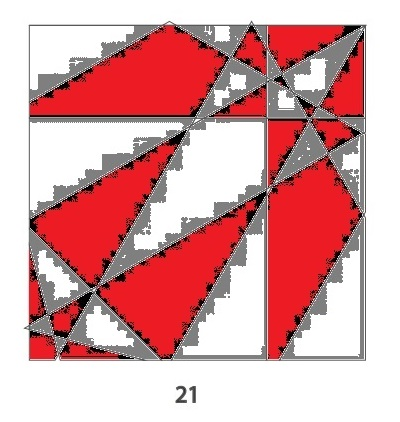I had so much fun doing this project. I have never considered myself to be much of an artist, but I felt a little artistic doing this project. I like all three tiles, but I think I like the final product the best.

You should join the project, too! You’re a star! Go ahead and shine! Whatever colors you use or whatever method you use to color your tile, your efforts will be appreciated. Also, because everyone is unique, every tile will look at least a little different. No tile will be ordinary.

Likewise, you might think that 729 is just an ordinary number, but much depends on how you look at it, too. Here are 729 equally sized squares.There’s a variety of ways to count the squares. Some ways remind us that 729 is a perfect square, other ways reminds us 729 is a perfect cube, and perhaps you can even find a way to see that 729 is a perfect 6th power, too.729 can be written as the sum of consecutive numbers several different ways:

• 364 + 365 = 729; that’s 2 consecutive numbers.
• 242 + 243 + 244 = 729; that’s 3 consecutive numbers.
• 119 + 120 + 121 + 122 + 123 + 124 = 729; that’s 6 consecutive numbers.
• 77 + 78 + 79 + 80 + 81 + 82 + 83 + 84 + 85 = 729; that’s 9 consecutive numbers.
• 32 + 33 + 34 + 35 + 36 + 37 + 38 + 39 + 40 + 41 + 42 + 43 + 44 + 45 + 46 + 47 + 48 + 49 = 729; that’s 18 consecutive numbers.
• 14 + 15 + 16 + 17 + 18 + 19 + 20 + 21 + 22 + 23 + 24 + 25 + 26 + 27 + 28 + 29 + 30 + 31 + 32 + 33 + 34 + 35 + 36 + 37 + 38 + 39 + 40 = 729; that’s 27 consecutive numbers.

729 is 1,000,000 in base 3, and 1000 in base 9, and 100 in base 27.

729 is also a palindrome in two bases:

• 1331 BASE 8; note that 1(512) + 3(64) + 3(8) + 1(1) = 729.
• 121 BASE 26; note that 1(26^2) + 2(26) + 1(1) = 729.

It is interesting that one less than 9 is 8, and one less than 27 is 26, and those are the two bases in which 729 is a palindrome!

Here is the factoring information for the number 729:

• 729 is a composite number. 729 = 27^2; 729 = 9^3; and 729 = 3^6
• Prime factorization: 729 = 3 x 3 x 3 x 3 x 3 x 3, which can be written 729 = (3^6)
• The exponent in the prime factorization is 6. Adding one we get (6 + 1) = 7. Therefore 729 has exactly 7 factors.
• Factors of 729: 1, 3, 9, 27, 81, 243, 729
• Factor pairs: 729 = 1 x 729, 3 x 243, 9 x 81, or 27 x 27
• 729 is a perfect square √729 = 27.512 Ants and Level 4The 512 Ants on Sullivan Street is an entertaining book for children that also includes learning activities by Marilyn Burns. The book teaches the important concept of doubling in a very natural way, but it can teach even more than that.  Here is a place value lesson for third grade students that uses the same book. There are relationships between adding, subtracting, doubling, multiplying, dividing, powers, and place value. The book and the place value lesson will make some of those relationships clear.

512 is a perfect cube because 512 = 8^3. It is also a cube of a cube because 8 = 2^3. Thus 512 = (2^3)^3 = 2^9. All of the factors of 512 are powers of 2.Print the puzzles or type the solution on this excel file: 12 Factors 2015-06-01

—————————————————————————————————

• 512 is a composite number.
• Prime factorization: 512 = 2 x 2 x 2 x 2 x 2 x 2 x 2 x 2 x 2, which can be written 512 = (2^9)
• The exponent in the prime factorization is 9. Adding one we get (9 + 1) = 10. Therefore 512 has exactly 10 factors.
• Factors of 512: 1, 2, 4, 8, 16, 32, 64, 128, 256, 512
• Factor pairs: 512 = 1 x 512, 2 x 256, 4 x 128, 8 x 64, or 16 x 32
• Taking the factor pair with the largest square number factor, we get √512 = (√256)(√2) = 16√2 ≈ 22.627417—————————————————————————————————343 and a Few Changes

Starting today, I’ve made a few small changes to the way my posts will look. If you can spot what the changes are, let me know if you have an opinion about them.

Quick Prime or Composite Number Test: If you divide 2^343 by 343, the remainder is 324, not 2, so 343 is NOT a prime number and is a composite number. (Use the mod function on the computer’s calculator to find the remainder.) Scroll down to see 343’s factors.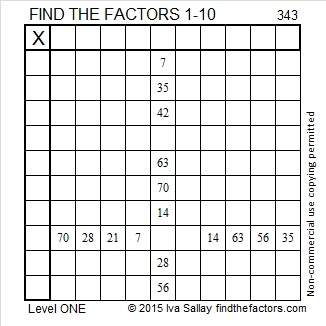Print the puzzles or type the factors on this excel file: 10 Factors 2015-01-05

• 343 is a composite number.
• Prime factorization: 343 = 7 x 7 x 7, which can be written 343 = 7³
• The exponent in the prime factorization is 3. Adding one, we get (3 + 1) = 4. Therefore 343 has exactly 4 factors.
• Factors of 343: 1, 7, 49, 343
• Factor pairs: 343 = 1 x 343 or 7 x 49
• Taking the factor pair with the largest square number factor, we get √343 = (√7)(√49) = 7√7 ≈ 18.520
• 343 is a perfect cube.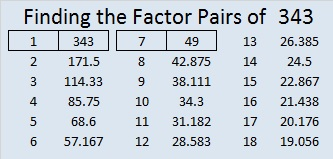(3+4)³ = 343, so 343 is a Friedman Number.